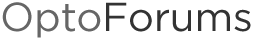# Polynomial Curve Equation

I have a table I have built for a air flow station that measures air velocity and outputs a deltaP reading. The table was built by manually taking pitot tube traverse measurements while using a PID loop to hold the deltaP output at several fixed setpoints. I plotted the results in excel and have a very nice curve and polynomial equation I would like to use.

y = -2E+06x6 + 3E+06x5 - 2E+06x4 + 610112x3 - 104541x2 + 14516x + 249.87

Where Y is the actual air velocity and x is the deltaP reading from the air flow station.

Can this be done? If so, how do I use the E function along with the ^

Normally I use a linear interpolation for something like this, but I think this method will yield more accurate results.Excellent question. FYI, easier programming of formulas/equations are the main reason we added OptoScript (years ago) into PAC Control…

Yes, use OptoScript (can be done in action blocks, but not recommended). The OptoScript code will look something like this:

``````//y = -2E+06x6           + 3E+06x5             - 2E+06x4             + 610112x3               - 104541x2               + 14516x      + 249.87;

y = -2.0e6 * Power(x, 6) + 3.0e6 * Power(x, 5) - 2.0e6 * Power(x, 4) + 610112.0 * Power(x, 3) - 104541.0 * Power(x, 2) + 14516.0 * x + 249.87;
``````

When doing formulas like this in OptoScript, a few recommendations:

[B]DO[/B] search the forums for code someone has already written and shared. For example, here’s some code to calculate Dew Point, Enthalpy, Wet Bulb which might save the next guy some time. Note that it came from some code / formulas found on the Internet. If you’re having trouble finding sample code on our website, [URL=“http://www.opto22.com/community/showthread.php?t=270”]click here for some search tips (especially Ben’s reply).

[B]DO[/B] use numbers like [B]2.0[/B] and not [B]2[/B] (that first coefficient) when you’re calculating a float. (You don’t want OptoScript to round to an integer if part of your formula looks like integers.)

[B]DO[/B] use lots of parenthesis so that the order of operations is obvious, especially for the people looking at the formula. Those don’t cost any thing and help make an ugly formula a little less-ugly.

[B]DON’T[/B] use variables like x and y. Variables can be up to 50 characters long and one-character variables are tricky to read and tricky to search for in your OptoScript. In this case, something like fActualAirVelocity and fDeltaP would be better (a little extra white space always helps too):

``````fActualAirVelocity =   -2.0e6 * Power(fDeltaP, 6)
+ 3.0e6 * Power(fDeltaP, 5)
- 2.0e6 * Power(fDeltaP, 4)
+ 610112.0 * Power(fDeltaP, 3)
- 104541.0 * Power(fDeltaP, 2)
+ 14516.0 * fDeltaP
+ 249.87;

``````

[B]DO[/B] test various values using your OptoScript vs. calculated by hand to make sure you got it right. If it’s not right the first time, break it down into pieces. Usually the problem is some kind of rounding error. (If that’s the case, check the types of your variables, and make sure you’re using values like 2.0 vs. 2, mentioned above, for float calculations.)

Here’s an example of how you might break down the equation above to step through in OptoScript when troubleshooting (in PAC Control Config mode, make sure have menu choice Configure > Full Debug selected):

``````
fPower6Piece =     -2.0e6 * Power(fDeltaP, 6);
fPower5Piece =      3.0e6 * Power(fDeltaP, 5);
fPower4Piece =     -2.0e6 * Power(fDeltaP, 4);
fPower3Piece =   610112.0 * Power(fDeltaP, 3);
fPower2Piece =  -104541.0 * Power(fDeltaP, 2);
fPower1Piece =    14516.0 * fDeltaP

y = fPower6Piece + fPower5Piece + fPower4Piece + fPower3Piece + fPower2Piece + fPower1Piece + 249.87;
``````

Finally, [B]DO[/B] share your working code on the forums and anything you learned along the way!

Hoping you’ll let us know how it goes…

-OptoMary